# Electricity Class 10 Important Questions Science Chapter 12

Solved the very best collection of Electricity Class 10 Science Important Questions and Answers Chapter 12 Pdf from the latest NCERT edition books, It will help you in scoring more marks in CBSE Exams.

## Electricity Class 10 Important Questions with Answers Science Chapter 12

### Electricity Class 10 Important Questions Very Short Answer Type

Question 1.
Write relation between heat energy produced in a conductor when a potential difference V is applied across its terminals and a current I flows through for ‘t’.   (CBSE 2013)
Heat produced, H = VIt.Question 2.
State difference between the wire used in the element of an electric heater and in a fuse wire.  (CBSE 2013)
The wire used in the element of electric heater has a high resistivity and have a high melting point, fuse wire have a low melting point and high resistivity.Question 3.
Name a device that helps to maintain a potential difference across a conductor.   (CBSE 2014)
Cell or battery.

Question 4.
Write S.I. unit of resistivity.   (CBSE 2015)
Ohm-metre (Ωm).

### Electricity Class 10 Important Questions Short Answer Type

Question 1.
Two wires of equal lengths, one of copper and the other of manganin (an alloy) have the same thickness. Which one can be used for:
(i) electrical transmission lines,
(ii) electrical heating devices? Why?  (CBSE 2008)
(i) Copper is used as electrical transmission lines as it has lesser resistivity.
(ii) Manganin is used for electrical heating divice as it has the comparatively higher resistivity, less oxidation and variation with temperature.

Question 2.
The following table gives the value of electrical resitivity of same materials:

 Material Copper Silver Constantan Electrical resistivity (in Ωm) 1.6 × 10-8 1.6 × 10-8 49 × 10-8

Which one is the best conductor of electricity out of them?   (CBSE 2008)
Silver and copper with less resistivity is the best conductor of electricity.

Question 3.
What is the effective resistance in the given circuit?   (AI 2008)R = R1 + R2 (Resistance in series)
∴ R = 2 + 2 = 4Ω.

Question 4.
Study the following circuit and answer the questions that follows: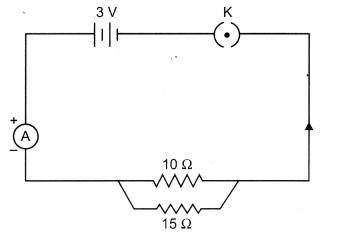(a) State the type of combination of the two resistors in the circuit.
(b) How much current is flowing through (i) 10 Ω and (it) 15 Ω resistor?
(c) What is the ammeter reading?   (AI 2008)
(a) Two resistors are in parallel combination.
(b) Current through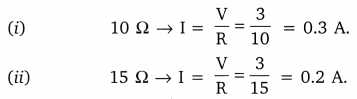(c) Ammeter reading = Total current flowing through the circuit = 0.5 A.

Question 5.
A wire is 1.0 m long, 0.2 mm in diameter and has a resistance of 10Ω. Calculate the resistivity of its material.  (CBSE 2009)
l = 1.0 m
d = 0.2 mm
R = 10Ω
p = ?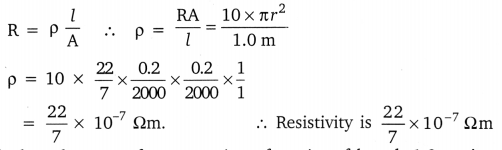Question 6.
Calculate the area of cross section of a wire of length 1.0 m, its resistance is 23 and the resistivity of the material of the wire is 1.84 x 10-6 dm.
R = 23Ω
l = 1.0 m
R = $$\rho \frac{l}{\mathrm{A}}$$
p = 1.84 × 10-6
A = ?
A = $$\frac{\rho l}{\mathrm{R}}$$
A = $$\frac{1.84 \times 10^{-6} \times 1}{23}$$ = 0.08 × 10-6 m2

Question 7.
Draw a schematic diagram of an electric circuit consisting of a battery of two cells each of 1.5 V, 5 Ω, 10 Ω and 15 Ω resistor and a plug key, all connected in series.   (AI 2009)Question 8.
Calculate resistance of an electric bulb which allows a 10 A current when connected to 220 V power source.  (AI 2009)
I = 10 A,
V = 220 V
∴ V = IR
R = ?
R = $$\frac{V}{I}=\frac{220}{10}$$ = 22Ω

Question 9.
A lamp rated 100 W at 220 V is connected to the mains electric supply. What current is drawn from the supply line if the voltage is 220 V?  (AI 2009)
P = 100 W
V = 220 V
P = VI
I = ?
∴ I = $$\frac{P}{V}=\frac{100}{220}=\frac{5}{11}$$ A.

Question 10.
Calculate the electric energy consumed by 120 W toaster in 20 minutes.   (AI 2009)
P = 120 W
t = 20 minutes
E = ?
E = P × t
E = 120 × 20 × 60 = 120 × 1200
E = 144 × 103 J.

Question 11.
Ten bulbs are connected in a series circuit to a power supply line and ten identical bulbs are connected in a parallel circuit to an identical power supply line.
(a) Which circuit would have the highest voltage across each bulb?
(b) In which circuit would the bulb be brighter?
(c) If one bulb blows out, in which circuit will other bulb stop glowing?
(d) Which circuit would have less current in it?
(a) Voltage is dependent on I and R. Hence, in parallel circuit.
(b) In parallel connection
(c) In series connection
(d) Series connection

Question 12.
How much current will an electric bulb draw from 220 V source if the resistance of the bulb is 1200 Ω If in place of bulb, a heater of resistance 100 Ω is connected to the sources, calculate the current drawn by it.   (CBSE 2012)
Given: V = 220 V,  R1 = 1200 Q,   I1 = ?,   R2 = 100 Ω,   I2 = ?
Using Ohm’s law,
V = I1R1Question 13.
Draw a schematic diagrams of an electric circuit comprising of 3 cells and an electric bulb, ammeter, plug-key in the ON mode and another with same components but with two bulbs in parallel and a voltmeter across the combination.   (CBSE 2012)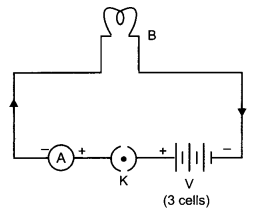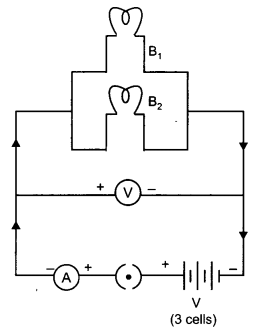Question 14.
Out of the two wires X and Y shown below, which one has greater resistance? Justify your answer.   (CBSE 2012)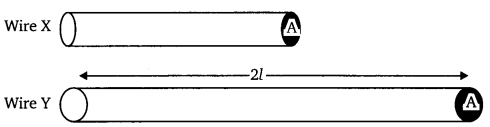Wire ‘Y has greater resistance as it has more length than wire ‘X’. It is because resistance of wire is directly proportional to the length of wire.

Question 15.
Two identical wires one of inchworm and other of copper are connected in series and a current (I) is passed through them. State the change observed in the temperatures of the two wires. Justify your answer. State the law which explains the above observation.    (CBSE 2012)
Nichrome wire has more resistivity than copper wire. Hence the large amount of heat is passed through nichrome wire.
(i) directly proportional to the square of current flowing through it, i.e., H ∝ I2.
(ii) directly proportional to the resistance offered by the conductor to the current, i.e., H ∝ R
(iii) directly proportional to the time for which current is flowing through it, i.e., H ∝ t
Combining these, we get
H ∝ I2Rt or H = KI2Rt
where K is proportionality constant and in SI system, it is equal to one.

Question 16.
An electric bulb is rated at 60 W, 240 V. Calculate its resistance. If the voltage drops to 192 V. calculate the power consumed and the current drawn by the bulb. (Assume that the resistance of the bulb remain unchanged.)  (CBSE 2012)
Given: P1 = 60 W,   V1 = 240 V,   R = ?,   P2 = ?,    V2 = 192 V,    I1 = ?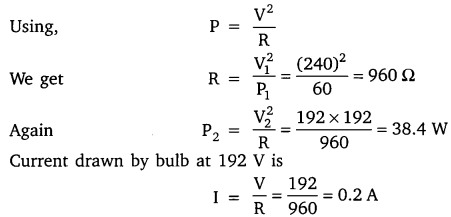Question 17.
Define 1 volt. Express it in terms of SI unit of work and charge. Calculate the amount of energy consumed in carrying a charge of 1 coulomb through a battery of 3V.  (CBSE 2014)
When 1 joule of work is done in carrying 1 coulomb of charge, from infinity to a point in the electric field, then potential at that point is called 1 volt. Potential difference between two points is
V = $$\frac{\mathrm{W}}{\mathrm{Q}}$$;
W = Q × V = 1 × 3 = 3J

Question 18.
V – I graph for two wires A and B are shown in the figure. If both wires are of same length and same thickness, which of the two is made of a material of high resistivity? Give justification for your answer.   (CBSE 2014)Greater the slope of V-I graph, greater will be the resistance of given metallic wire. In the given graph, wire A has greater slope than B. Hence, wire A has greater resistance.
For the wires of same length and same thickness, resistance depends on the nature of material of the wire,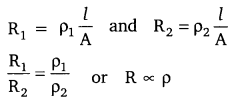Hence, wire A’ is made of a material of high resistivity.

Question 19.
(i) Draw a closed circuit diagram consisting of a 0.5 m long nichrome wire XY, an ammeter, a voltmeter, four cells of 1.5 V each and a plug key.
(ii) Following graph was plotted between V and I values:
What would be the values of V/I ratios when the potential difference is 0.8 V, 1.2 V and 1.6 V respectively? What conclusion do you draw from these values?   (CBSE 2015)(i)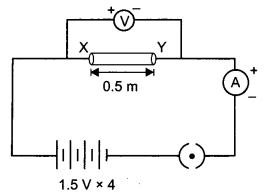(ii) From the graph, when p.d. is 1.6 volt and 0.6 A current.
$$\frac{V}{I}=\frac{1.6}{0.6}=2.67 \Omega$$
Therefore, value of $$\frac{\mathrm{V}}{\mathrm{I}}$$ ratio for all potential differences of 0.8 V,  1.2 V and 1.6 volt will be equal to 2.67 Ω.
We conclude that at the given temperature, the resistance of wire is constant and is equal to 2.67 Q.

Question 20.
Study the following electric circuit and find
(i) the current flowing in the circuit and
(ii) the potential difference across 10 Ω resistor.    (CBSE 2015)10 Ω and 20 Ω are connected in series, their equivalent resistance is
Rs = R1 + R2 = 10 + 20 = 30 Ω
(i) Current flowing in the circuit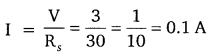(ii) Potential difference across 10 resistor
V = IR = $$\frac{1}{10}$$ × 10 = 1 volt.

Question 21.
Find the current drawn from the battery by the network of four resistors shown in the figure.   (CBSE 2015)Equivalent resistance of the given network is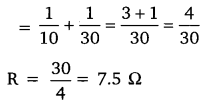Current drawn from the battery
$$I=\frac{V}{R}=\frac{3}{7.5}=\frac{30}{75}=\frac{2}{5}$$
I = 0.4 A

### Electricity Class 10 Important Questions Long Answer Type

Question 1.
For the circuit shown in the diagram given below:
Calculate:
(a) the value of current through each resistor.
(b) the total current in the circuit.
(c) the total effective resistance of the circuit.   (AI 2008)Data R1 = 5
V = 6 V
R2 = 10Ω     I2 = ? (through each resistor)
R3 = 30Ω     I3 = ? (total)
R Total = ?
(a)(b) Total current
I = I + I10Ω + I30Ω.
I = 1.2 + 0.6 + 0.2 = 2.0 A.

(c)
$$\frac{1}{\mathrm{R}_{\mathrm{P}}}=\frac{1}{\mathrm{R}_{1}}+\frac{1}{\mathrm{R}_{2}}+\frac{1}{\mathrm{R}_{3}}$$Rp = 3

Question 2.
(a) What is meant by saying that potential difference between two points is 1 volt? Name a device that helps to measure the potential difference across a conductor.
(b) Why does the connecting cord of an electric heater not glow hot while the heating element does?
(c) Electrical resistivities of some substances at 20°C are given below:
Silver   1.6 × 10-8 Ωm
Copper   1.62 × 10-8 Ωm
Tungsten   5.20 × 10-8 Ωm
Iron   10.0 × 10-8 Ωm
Mercury   94.0 × 10-8 Ωm
Nichrome   100 × 10-6 Ωm.
Answer the following questions in relation to them:
(i) Among silver and copper, which one is better conductor?
(ii) Which material would you advice to be used in electrical heating devices? Why?   (CBSE 2008)
(a) Potential difference is 1 volt means the work done in carrying a unit positive charge from one point to another point is 1 Joule. Voltmeter is used to measure p.d.

(b) The heating element offers higher resistance to the flow of electrons in it and glows when the current passes through it but the cord offers extremely low resistance.

(c) (i) Silver is better conductor as its resistivity is less than copper.
(ii) Nichrome alloy can be used to make heating devices as they do not oxidise or burn at high temperature and offers higher resistance to the flow of current so as to convert electric energy into heat energy.

Question 3.
(a) Name an instrument that measures electric current in a circuit. Define the unit of electric current.
(b) What do the following symbols mean in circuit diagram?
(i)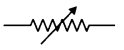(ii)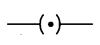(c) An electric circuit consisting of a 0.5 m long nichrome wire, an ammeter, a voltmeter, four cells of 1.5 V each and a plug key was set up.
(i) Draw a diagram of the electric circuit to study the relation between the potential difference maintained between the points ‘X’ and ‘Y’ and the electric current flowing through XY.
(ii) Following graph was plotted between V and I values: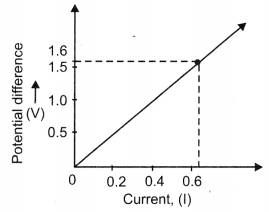What would be the values of $$\frac{V}{I}$$ ratios when the potential difference is 0.8 V, 1.2 V and 1.6 V respectively? What conclusion do you draw from these values?   (AI 2008)
(a) Ammeter is used to measure electric current. Its S.I. units is ampere, it is connected in series. One ampere is defined as the current which will flow in a resistor of 1 Ω, when 1 volt potential difference is applied across its ends.

(b)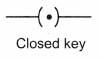(c) (i) Circuit diagram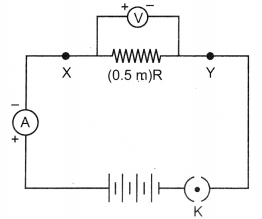(ii) V = 1.6 V
I = 0.6 A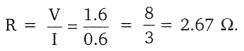V I = V/R V/I 0.8 V 0.8/2.67 = 0.30 8/3 1.2 V 1.2/2.67 = 0.45 8/3 1.6 V 1.6/2.67 = 0.60 8/3

$$\frac{V}{I}$$ ratios are constant for different V and I values.
Conclusion: ‘R’ is constant at given temperature. VI graph shows straight line.

Question 4.
In the circuit diagram given below: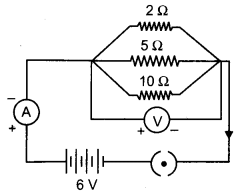Calculate:
(a) the current through each resistor.
(b) the total current in the circuit.
(c) the total effective resistane of the circuit.    (CBSE 2008)
(a) Current through 2 Ω resistor I1 = $$\frac{V}{R}=\frac{6}{2}$$ = 3 A.
Current through 5 Ω resistor I2 = $$\frac{V}{R}=\frac{6}{5}$$ =1.2 A.
Current through 10 Ω resistor I3 = $$\frac{V}{R}=\frac{6}{10}$$ = 0.6 A.

(b) Total current in the circuit = 3 + 1.2 + 0.6 = 4.8 A
(c) Total resistance, in parallel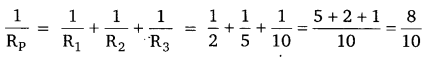Rp = $$\frac{10}{8}$$ = 1.25

Question 5.
Two lamps, one rated 60 W at 220 V and the other 40 W at 220 V, are connected in parallel to the electric supply at 220 V.
(a) Draw a circuit diagram to show the connection.
(b) Calculate the current drawn from the electric supply.
(c) Calculate the total energy consumed by two lamps together when they operate for one hour. (CBSE 2008)
(a)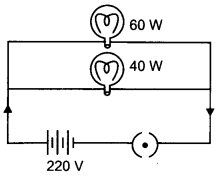(b) Current drawn by 40 W lamp
P =VI
∴ I = $$\frac{P}{V}=\frac{40}{220}=\frac{2}{11}$$A.
Current drawn by 60 W lamp P =VI
P = VI
∴ I = $$\frac{P}{V}=\frac{60}{220}=\frac{3}{11}$$A.
Total current drawn = $$\frac{2}{11}+\frac{3}{11}=\frac{5}{11}$$ A = 0.45 A

(c) Total energy consumed in one hour by both the lamps
E = P × t
∴ E = (40 + 60 W) × 1 hour
= 100 W × 1 hour
= 0.1 kW × 1 hour = 0.1 kWh.

Question 6.
A piece of wire of resistance 20 Ω is drawn out so that its length is increased to twice its original length. Calculate the resistance of the wire in the new situation.   (CBSE 2009)∴ R2 = 2 R1 (R1 = 20 Ω) = 2 × 20 Ω = 40 Ω.
∴ Resistance in new case = 40 Ω.

Question 7.
Two resistors with resistance 5 Ω and 10 Ω respectively are to be connected to a battery of emf 6 V so as to obtain:
(i) minimum current flowing,
(ii) maximum current flowing:
(a) How will you connect the resistance in each case?
(b) Calculate the strength of the total current in the circuit in the two cases.   (CBSE 2009)
(a) (i) To get minimum current – Resistance will be connected in series
(ii) To get maximum current the resistance should be connected in parallel.

(b) Strength of current in series
R = R1 + R2 = 5 + 10 = 15 Ω
V = 6V
∴ I = $$\frac{V}{R}=\frac{6}{15}$$
Strength of current in parallel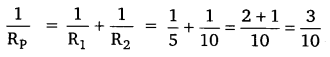Rp = $$\frac{10}{3}$$
V = 6V
∴ I = $$\frac{V}{R}=\frac{6}{10 / 3}=\frac{18}{10}$$ = 1.8 A

Question 8.
(a) Define the term ‘Volt’.
(b) State the relation between work, charge and potential difference for an electric circuit.
Calculate the potential difference between the two terminals of a battery if 100 joules of work is required to transfer 20 coulombs of charge from one terminal of the battery of the other.   (AI 2009)
(a) Volt is defined as the work done in Joules, in carrying a coulomb of charge from one point to another.

(b) Work = W (J)
Charge = Q (C)
V = $$\frac{W}{Q}$$
Potential difference = V
1V = $$\frac{1J}{1C}$$
W = 100 J; Q = 20 C; V = ?
∴ V = $$\frac{W}{Q}=\frac{100}{20}$$ = 5 Volt
∴ Potential difference = 5 V

Question 9.
(a) Though same current flows through the electric line wires and the filament of bulb, yet only the filament glows. Why?
(b) The temperature of the filament of bulb is 2700°C when it glows. Why does it not get burnt up at such high temperature?
(c) The filament of an electric lamp, which draws a current of 0.25 A is used for four hours. Calculate the amount of charge flowing through the circuit.
(d) An electric iron is rated 2 kW at 220 V Calculate the capacity of the fuse that should be used for the electric iron. (CBSE 2012)
(a) Electric line wires offer extremely low resistance to the flow of current, so they do not glow because negligible heat is produced in it. The filament of bulb glows because it becomes red hot due to large amount of heat produced, as it offers high resistance to the flow of current through it.

(b) The filament of bulb when it glows at 2700 °C does not get burnt because the tungsten metal of filament has
(i) a very high melting point (of 3380 °C) and
(ii) a high resistivity.

(c) Given: I = 0.25 A, t = 4 h = 4 × 60 × 60 sec.
So, amount of charge flowing the filament of electric lamp
q = It = 0.25 × 4 × 60 × 60 = 3600 C

(d) Given: P = 2kw = 2000 W, V = 220 V
Using, P = VI
2000 = 220 × 1
I = $$\frac{2000}{220}$$ = 9.09 A

(c) I = 0.25 A, t = 4h = 4 × 60 × 60 sec.
Amount of charge flowing the filament of electric lamp the capacity of the fuse that should be used for the electric iron is of the order or 10 A.

Question 10.
(a) Calculate the resistance of 1 km long copper wire of radius 1 mm. Resistivity of the copper is 1.72 × 10-8 fim.
(b) Draw a schematic diagram of a circuit consisting of a battery of 4 cells of 2V each connected to a key, an ammeter and two resistors of 2 Ω and 3 Ω respectively in series and a voltmeter to measure potential difference across 3 Ω. (CBSE 2013)
(OR)
When a high resistance voltmeter is connected directly across a resister its reading is 2 V An electric cell is sending the current of 0.4 A, (measured by an ammeter) in the electric circuit in which a rheostat is also connected to vary the current.
(a) Draw an equivalent labeled circuit for the given data.
(b) Find the resistance of the resistor.
(c) Name and state the law applicable in the given case. A graph is drawn between a set of values of potential difference (V) across the resistor and current (I) flowing through it. Show the nature of graph thus obtained.
(a) L = 1 km = 1000 m
R = 1 mm = 1 × 10-3 m
A = πr2 = 3.14 × (1 x 10-3)2
ρ = 1.72 × 10-8 Ωm.
Resistance of the wire R = $$\rho \frac{\mathrm{L}}{\mathrm{A}}$$
R = 1.72 × 10-8 × $$\frac{1000}{3.14 \times\left(10^{-3}\right)^{2}}$$
R = 5.5 Ω

(b)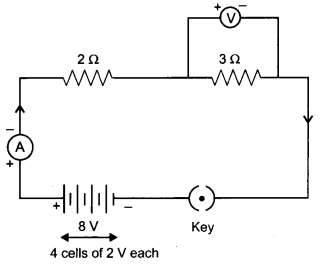OR
(a)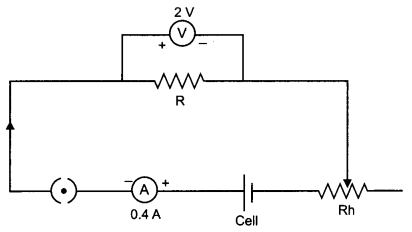(b) Resistance of resistor is R = $$\frac{V}{I}=\frac{2}{0.4}$$ = $$\frac{20}{4}$$ = 5

(c) Ohm’s law: When the physical conditions such as temperature remain same, the current flowing through the conductor is directly proportional to the potential difference applied across the ends of the conductor.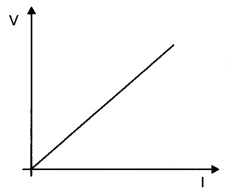The graph between the V and I would be linear i.e., straight line.

Question 11.
Two wires A and B are of equal length and have equal resistance. If the resistivity of A is more than that of B which wire is thicker and why? For the electric circuit given below calculate: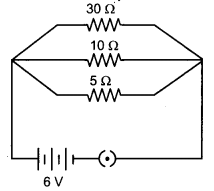(i) Current in each resistor,
(ii) Total current drawn from the battery, and
(iii) Equivalent resistance of the Circuit.   (CBSE 2014)
R = $$\rho \frac{\mathrm{L}}{\mathrm{A}}$$
$$\frac{\rho}{A}=\frac{R}{l}$$ = Constant = K (for same l and R)
ρ = kA
ρ ∝ A
So, for different materials having same resistance per unit length, greater resistivity material wire has more cross-sectional area.
(i) Current through each resistorCurrent in 5 Ω, 10 Ω and 30 Ω are therefore, 1.2 A, 0.6 A and 0.2 A respectively.

(ii) Total current drawn from the battery
I = I1 + I2 + I3 = 1.2 + 0.6 + 0.2 = 2.0 A

(iii) R1 = 5 Ω, R2 = 10 Ω and R3 = 30 Ω are connected in parallel. So their equivalent resistance.R = 3 Ω

Question 12.
(a) Define electric power. Express it in terms of potential difference V and resistance R.
(b) An electrical fuse is rated at 2 A. What is meant by this statement?
(c) An electric iron of 1 kW is operated at 220 V Find which of the following fuses that respectively rated at 1 A, 3 A and 5 A can be used in it.   (CBSE 2014)
(a) Electric power: It is the rate of doing work by an energy source or the rate at which the electrical energy is dissipated or consumed per unit time in the electric circuit is called electric power.
So,(b) It means, the maximum current will flow through it is only 2 A. Fuse wire will melt if the current exceeds 2 A value.

(c) Given : p = 1 kW = 1000 W,  V = 220 V
current drawn, I = $$\frac{P}{V}=\frac{1000}{220}=\frac{50}{11}$$ = 4.54 A
To run electric iron of 1 kW, rated fuse of 5 A should be used.

Question 13.
What is meant by electric current? Name and define its SI unit. In a conductor electrons are flowing from B to A. What is the direction of conventional current? Give justification for your answer.
A steady current of 1 ampere flows through a conductor. Calculate the number of electrons that flows through any section of the conductor in 1 second. (Charge on electron 1.6 x 10-19 coulomb).    (CBSE 2015)